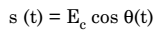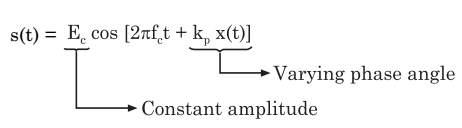# What is Phase Modulation

## Phase Modulation (PM)

Phase modulation is a type of angle modulation in which the angular argument θ(t) is changed in linear proportion with the instantaneous magnitude of the message signal x(t).

Here, it may be noted that the first term in the above expression i.e., 2πfct represents the angular argument of the unmodulated carrier (i.e., when x (t) = 0).

Whereas the second term kp x (t) represents the proportional phase change, because kp is the phase sensitivity in radians per volt.

x (t) is the message signal which is assumed to be a voltage signal.

The value of fc i.e., θ(t) at t = 0 has been assumed to be equal to zero for the sake of convenience.

### Mathematical Expression for PM

A phase modulated can be mathematically expressed in time domain as follows:Substituting the expression for θ(t), we get,

PM Wave :This expression shows that the PM wave has a constant amplitude equal to that of the carrier but the phase shift is a function of x (t).

We can also note that there is a proportional change in frequency associated with the phase change.